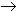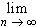#Interactive Real Analysis

Next | Previous | Glossary | Map

## 7.2. Integration Techniques

### Example 7.2.7: Integration by Parts and Limits

Suppose f:[a, b]R is a continuously differentiable function. Show that:
1.f(x) sin(nx) dx = 0
2.f(x) cos(nx) dx = 0

We already mentioned that this will be an application of integration by parts. Let's focus on the first statement and let g'(x) = sin(nx), where g' is the function in the Integration by Parts theorem. Then:f(x) sin(n x) dx = - 1/n cos(nx) f(x) + 1/nf'(x) cos(nx) dx

But |sin(nx)|1 and |cos(nx)|1, and since both f and f' are continuous functions on a closed, bounded interval there are constants K and L such that |f(x)|K and |f'(x)|L. Putting everything together we get:

|f(x) sin(n x) dx |K/n + L(b-a)/n

Thus,f(x) sin(n x) dx = 0
The proof of the second statement is left as an exercise.
Next | Previous | Glossary | Map